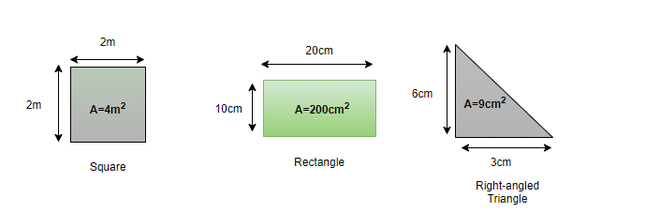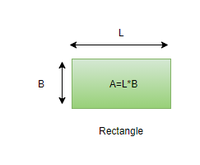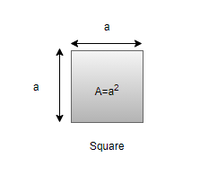# What is the formula for area of a rectangle and square?

• Last Updated : 18 Oct, 2021

Mensuration is a Greek word that means ‘measurement’. Mensuration is a branch of mathematics that involves the calculation of geometric figures like squares, rectangles, cones, cylinders, etc. With the help of mensuration, one can learn how to calculate the area, parameter, surface area, etc. which are the fundamentals of almost every calculation in the field of Mathematics. Now, Let’s begin with the most basic formula of the mensuration i.e. Area. Let’s first understand what an area is.

### Area

The area is the size of a surface or the amount of space occupied by a closed region. It is calculated for 2D figures and is expressed in units like m2, cm2, etc. The unit of area is always a square unit. It is denoted with the alphabet A.  The diagram below shows examples of geometric figures along with their calculated area,

Attention reader! All those who say programming isn't for kids, just haven't met the right mentors yet. Join the  Demo Class for First Step to Coding Coursespecifically designed for students of class 8 to 12.

The students will get to learn more about the world of programming in these free classes which will definitely help them in making a wise career choice in the future.Lets now look into the formula for the calculation of the area of the rectangle

### What is the formula for the area of a square and rectangle?

The rectangle is a geometric shape with four sides whose each angle is 90°. Out of the four sides, the two sides of the rectangle are equal and parallel with one side longer than the other. A rectangle looks like as shown in the below figure:Area of Rectangle

In the above figure, the longer side is the length and the shorter side is the breadth of the rectangle.

Formula

The formula for calculating the area of a rectangle can be derived with the help of the below-mentioned steps:

1. The length and breadth (Width) of the rectangle should be known in advance.
2. Length and breadth are multiplied and the result obtained is the required area.
3. The unit of area is square of the unit of its length and breadth

From the above steps, the formula of a rectangle can be written like this.

Area of a rectangle (A) = Length(L) × Breadth(B), where L is the length of the rectangle and B is the breadth of the rectangle.

Note If the unit of length and breadth is not the same then it should be converted to one unit. For e.g. If the length is in cm and breadth in m then both the side should be changed either to m or cm.

Properties of a rectangle

1. The opposite sides of the rectangle are equal and parallel.
2. The diagonal is of the same length.
3. All the angles are 90°.
4. The diagonals of a rectangle bisect each other.

#### Square

Square is a geometric shape with all four sides of equal length. Each side makes an angle of 90°. All four sides of the square are equal and parallel. A square is the same as a rectangle, the only difference being that all sides in a square are equal. A square looks like as shown in the figure below:Area of Square

Formula

The formula for calculating the area of a square can be written like this:

Area of square (A) = Length = Breadth = a × a = a2 where, a is the side of the square.

Properties of the square

1. All sides of the square have equal length.
2. The angles of each side is equal i.e. 90 degree
3. The opposite sides of the square are parallel.
4. The length of the diagonals is equal.

### Sample Problems

Question 1: Find the area of a rectangle with a length of 5cm and a breadth of 2 cm.

Solution:

Given,

Length = 5cm

Formula:

A = 5cm × 2cm = 10cm2

Question 2: Find the area of a rectangle whose length and breadth are 10cm and 0.5m respectively.

Solution:

Given,

Length = 10cm

Let us first change the breadth in cm

1m = 100cm

Breadth = 0.5 × 100 = 50cm

Formula:

A = Length × Breadth = 10 × 50 = 500cm2

Question 3: Find the area of a square whose side is 4m.

Solution:

Given, a = 4m

A = a2 = (4)2 = 16m2

Question 4: Find the area of a square plot of the side 32cm.

Solution:

Given, a = 32cm

A = a2 = (32)2 = 1024cm2

My Personal Notes arrow_drop_up